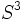# Group of unit quaternions

View a complete list of particular groups (this is a very huge list!)[SHOW MORE]

## Definition

This group is denoted$S^3, SU(2), S^0(\mathbb{H}), S^1(\mathbb{C})$ and is defined in a number of equivalent ways.

### As the group of unit quaternions

Denote by$\mathbb{H}$ the division ring of Hamiltonian quaternions. The group we are interested in is the multiplicative subgroup of$\mathbb{H}^*$ comprising those unit quaternions$a + b\mathbf{i} + c\mathbf{j} + d\mathbf{k}$ satisfying$a^2 + b^2 + c^2 + d^2 = 1$. Note that$a,b,c,d \in \R$ (and are allowed to be equal). Explicitly, the multiplication is given by:$(a_1 + b_1\mathbf{i} + c_1\mathbf{j} + d_1\mathbf{k})(a_2 + b_2\mathbf{i} + c_2\mathbf{j} + d_2\mathbf{k}) = (a_1a_2 - b_1b_2 - c_1c_2 - d_1d_2) + (a_1b_2 + a_2b_1 + c_1d_2 - c_2d_1)\mathbf{i} + (a_1c_2 + a_2c_1 + d_1b_2 - d_2b_1)\mathbf{j} + (a_1d_2 + a_2d_1 + b_1c_2 - b_2c_1)\mathbf{k}$

The identity element is:$1 + 0\mathbf{i} + 0\mathbf{j} + 0\mathbf{k}$

The inverse is given by:$(a + b\mathbf{i} + c\mathbf{j} + d\mathbf{k})^{-1} = a - b\mathbf{i} - c\mathbf{j} - d\mathbf{k}$

### As the special unitary group

The group can also be defined as the special unitary group of degree two over the field of complex numbers. It is denoted$SU(2)$ or$SU(2,mathbb{C})$.

### Structures

The group has the following structures:

## Arithmetic functions

Function Value Similar groups Explanation
dimension of an algebraic group 3 As$SU(n,\mathbb{C}), n = 2$:$n^2 - 1 = 2^2 - 1 = 3$As$S^n, n = 3$:$n = 3$ (note that$S^n$ is a group only for$n = 0,1,3$)
dimension of a real Lie group 3 As$SU(n,\mathbb{C}), n = 2$:$n^2 - 1 = 2^2 - 1 = 3$
As$S^n, n = 3$:$n = 3$ (note that$S^n$ is a group only for$n = 0,1,3$)

## Group properties

### Abstract group properties

Property Satisfied? Explanation
abelian group No Follows from center of general linear group is group of scalar matrices over center
nilpotent group No Follows from special linear group is quasisimple
solvable group No Follows from special linear group is quasisimple
simple group No Has a proper nontrivial center, also has normal subgroup$SL(2,\R)$.
almost simple group No Has a nontrivial center.
quasisimple group Yes It is a perfect group and its quotient by its center is SO(3,R) which is simple.
almost quasisimple group Yes Follows from being quasisimple.
divisible group Yes For any element of the group and any natural number$n$, the element has a$n^{th}$ root in the group.

### Topological/Lie group properties

The topology here is the subspace topology from the Euclidean topology on the set of all matrices, which is identified with the Euclidean space$\R^4$.

Property Satisfied? Explanation
connected topological group Yes easy to see from geometric description as$S^3$.
compact group Yes Easy to see from geometric description as$S^3$.